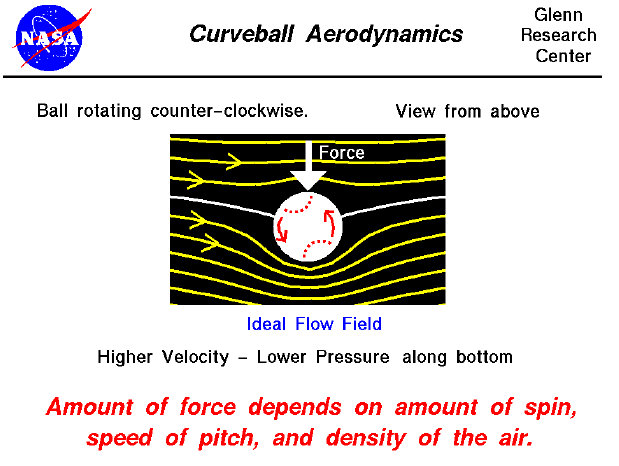All that is necessary to create lift is to turn a flow of air. An airfoil of a wing will turn a flow, but so will a spinning ball. The exact details are fairly complex and are given on a separate slide. Summarizing the results, the amount of force generated by a spinning ball will depend on the amount of spin, the velocity of the ball (or fluid), and the density of the fluid.

The slide shows a view of the flow as if we were moving with the ball looking down from above. The ball appears stationary, and the flow moves from left to right. As the ball spins, the air near the surface of the ball moves with the surface of the ball. If there was no free stream flow and the ball was stopped and spinning, there would be circular flow around the ball which would match the speed of rotation at the surface and die away to nothing far from the ball. When the free stream flow is added to this circular flow, the resulting flow has a net turning and produces a force. On this figure the ball spins counterclockwise, so the free stream flow over the top of the ball is opposed by the circular flow; the free stream flow below the ball is assisted by the circular flow. In the figure we can see that the net streamlines around the ball are distorted because of the spinning. The net turning of the flow has produced a downward force.

As the force acts on the ball, it will be deflected along it's flight path. The mathematical details of the ball's trajectory are given on a separate slide.

(Be particularly aware of the simplifying assumptions that have gone into this analysis. The type of flow field shown in the figure is called an ideal flow field. We have produced the ideal flow field by superimposing the flow field from an ideal vortex centered on the ball with a uniform free stream flow. There is no viscosity in this model, no boundary layer on the ball, even though this is the real origin of the circulating flow! In reality, the flow around a spinning baseball is very complex. The ball isn't even smooth; the stitches used to hold the covering together stick up out of the boundary layer. In addition, the flow off the rear of the ball is separated and can even be unsteady. BUT, the simplified model does give the first order effects--it gives an initial good prediction of the motion of the ball.)

Guided Tours